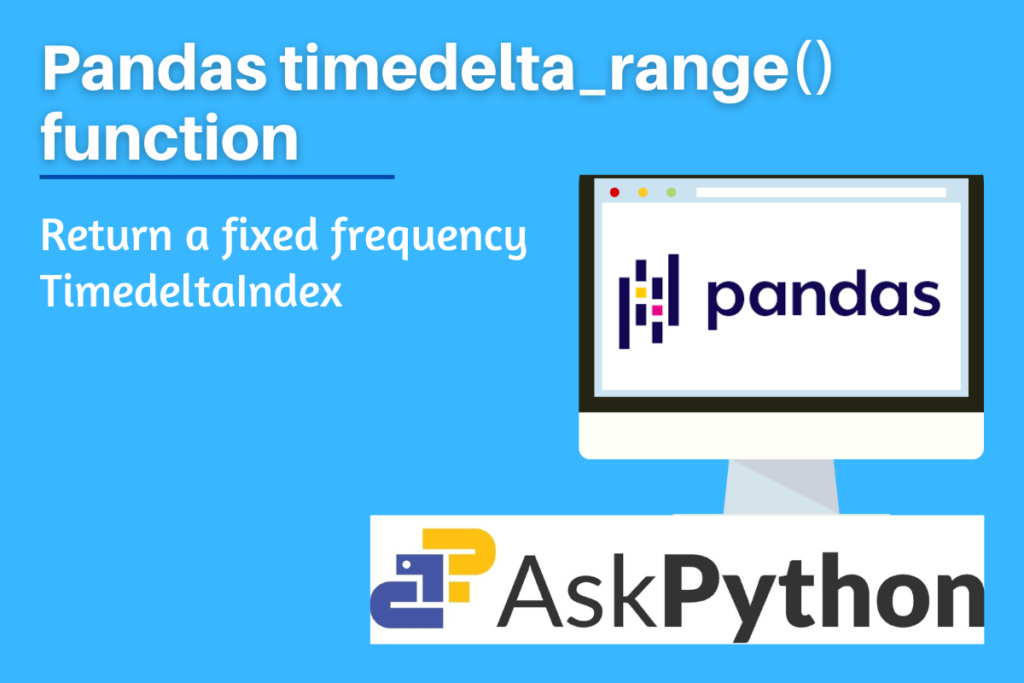# Pandas timedelta_range – Return a fixed frequency TimedeltaIndexIn this post, let us try to learn how to use the `timedelta_range()` function, one of the Pandas package’s general functions. To modify and understand data, the Python computer language’s Pandas software package is employed. The Pandas package The terms “Pandas” are intended to refer to both “Panel Data” and “Python Data Analysis.” It offers specific approaches and data structures for working with time series and mathematical as well as statistical tables. It is an open-source package and works great on real-world unorganized and enormous data. These features of this package make it the best tool for data scientists and data analysts.

One such function to work on time series is the `timedelta_range() `function of the Pandas package. It helps to create customized series of timedeltaIndex. Let’s understand its syntax and implementation in python.

Also read: Pandas to_timedelta – Convert argument to timedelta.

## Why is timedelta_range used?

This function provides a fixed frequency TimedeltaIndex with the default being day. Exact three of the four parameters start, end, periods, and freq must be given. The resultant TimedeltaIndex will have linearly separated values between the start and end of the frequency left out (closed on both sides).

Also, check out the period_range() function of the Pandas package. It is very similar to the function we are discussing. The only difference is that it returns a fixed frequency in PeriodIndex format.

### Syntax of Pandas timedelta_range

```pandas.timedelta_range(start=None, end=None, periods=None, freq=None, name=None, closed=None)
```
• start: string or timedelta-like, default is set to ‘None’
• For creating timedeltas, this value is used as a left bound.
• end: string or timedelta-like, default is set to ‘None’
• For creating timedeltas, this value is used as a right bound.
• periods: integer, default is set to None
• The number of periods to produce.
• freq: string or DateOffset, default is set to ‘D’
• Multiple frequency strings are possible, like “3H.”
• name: string, default is set to ‘None’
• Resulting TimedeltaIndex’s name.
• closed: str, default is set to None
• Close the interval to either the left, right, or both sides of the specified frequency (None).

## Implementing Pandas timedelta_range

Before beginning the methods, be sure to install and load the pandas package into your IDE. Run the following line of code in your IDE to accomplish this.

```import pandas as pd
```

### Example 1: Passing the periods parameter

```x = pd.timedelta_range(start='1 day', end='5 days', periods=3)
y = pd.timedelta_range(start='1 day', periods=3)
z = pd.timedelta_range(end='10 days', periods=3)
print(x, "\n\n", y, "\n\n", z)
```

Note: When all three parameters ‘start’, ‘end’ and ‘periods’ are mentioned, then the frequency is automatically generated (linearly spaced).

### Example 2: Passing the frequency ‘freq’ parameter

```x = pd.timedelta_range(start='1 day', end='4 days', freq='2D')   #frequency set to 2 days
y = pd.timedelta_range(start='1 day', end='4 days', freq='10H')  #frequency set to 10 hours
z = pd.timedelta_range(start='1 day', end='4 days', freq='1.5D') ##frequency set to one and a half day i.e 36hrs
print(x, "\n\n", y, "\n\n", z)
```

Note that in the last example- the ‘z’ one, frequency is set as 1.5 day which is automatically converted into 36 hours.

### Example 3: Passing the closed parameter

```x = pd.timedelta_range(start='1 day', end='7 days', periods=3)  #default closed set to none
y = pd.timedelta_range(start='1 day', end='7 days', periods=3, closed='right')
z = pd.timedelta_range(start='1 day', end='7 days', periods=3, closed='left')
print(x, "\n\n", y, "\n\n", z)
```